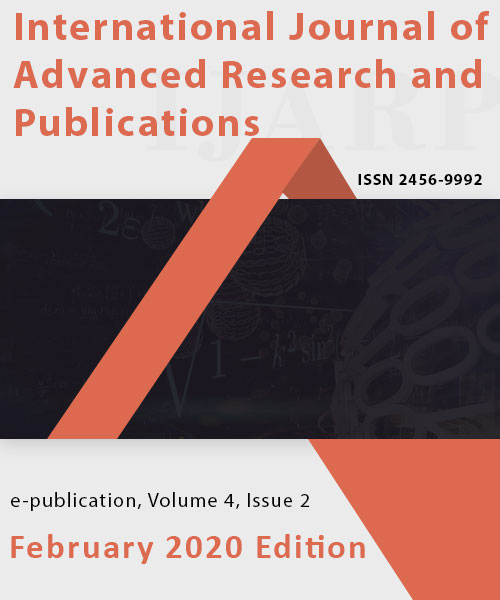# International Journal of Advanced Research and Publications

## High Quality Publications & World Wide Indexing!

#### A Cauchy Problem For A Class Of Quasi-Linear Hyperbolic Second Order Partial Differential Equation (PDE) In A Banach Space.Volume 4 - Issue 2, February 2020 Edition

Author(s)
O. Echude, M. T. Y. Kadzai, B. Balarabe, H. Mohammed
Keywords
Accretive, Cauchy Problem, Quasi-linear PDE, Banach Space
Abstract
A class of quasi-linear hyperbolic second order partial differential equation (PDE) in a Banach space was investigated using some fundamental result established by Browder and Egwurube. The quasi-linear hyperbolic second order partial differential equation was converted to Cauchy-like equation, than show that the operator A is accretive, M-accretive and thus admits a solution.
References
 B. L., Rozhdestvenskii, (2001) , "Non-linear partial differential equation", in Hazewinkel, Michiel, Encyclopedia of Mathematics, Springer Science+Business Media B.V. / Kluwer Academic Publishers, ISBN 978-1-55608-010-4

 E. Levi, "Sur problema di Cauchy per le equazioni a caratteristiche reali e distinti" Rend. R. Acad. Lincei (5), 17 1908, pp 331-339

 D. K. Gvazava (originator), Quasi-linear hyperbolic equations and systems. Encyclopedia of Mathematics. http://www.encyclopediaofmath.org/index.php?title=Quasi-linear_hyperbolic_equations_and_systems&oldid=16213

 M. O. Egwurube, & E. J. D. Garba, â€œA Cauchy problem for a quasi-linear hyperbolic first-order partial differential equationâ€. Journal of Nigeria Association of Mathematical Physics, 7, 29 â€“ 32. 2003.

 A. U. Bawa, M. O. Egwurube, & M. M. Ahmad, â€œA quasi-linear parabolic partial differential equation with accretive propertyâ€. Tanzania Journal of Natural and Applied Sciences. 3, 433-438, 2012.

 S. I. Pokhozhaev (originator) Non-Linear Partial Differential Equation. Encyclopedia of Mathematics. URP: http://www.encyclopediaofmath.org/in dex.php?title=Non-linear_hyperbolic_ equations_and_systems&oldid=28254

 F. E. Browder, â€œNon-linear mappings of non-expansive and accretive type in Banach spaceâ€. Bulletin of the American Mathematical Society, 73, 875 â€“ 882, 1967.

 F. E. Browder, â€œNon-linear operators and nonlinear equations of evolutions in Banach spacesâ€, Non-linear functional analysis, Proceedings of the Symposia on Pure Mathematics,American Mathematical Society,. 18, Part 2, 1970